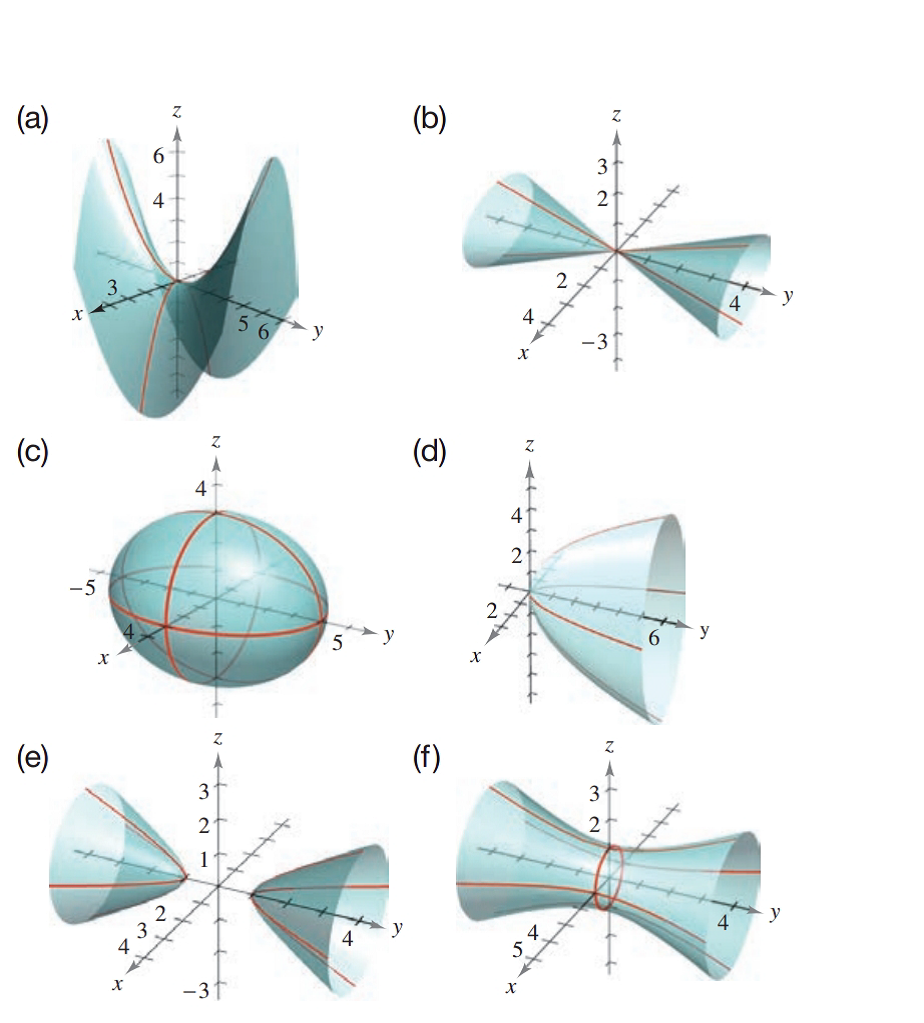Chapter 7.2, Problem 28E### Calculus: An Applied Approach (Min...

10th Edition
Ron Larson
ISBN: 9781305860919

#### Solutions

Chapter
Section### Calculus: An Applied Approach (Min...

10th Edition
Ron Larson
ISBN: 9781305860919
Textbook Problem
5 views

# Matching In Exercises 23-28, match the equation with its graph. [The graphs are labeled (a)-(f).]4 x 2 − y 2 + 4 z = 0

To determine

The quadratic surface represented by the equation 4x2y2+4z=0 and its correct graph from the provided graph.

Explanation

Given Information:

The provided quadratic surface equation is 4x2y2+4z=0 and the provided option for matching as follows:

Consider the provided equation 4x2y2+4z=0

Rewrite the equation as in standard format,

4x2y2+4z=04x24y24=4z4x21y24=4z4x21y24=2z4

The above equation can be written as,

x2(1)2y

### Still sussing out bartleby?

Check out a sample textbook solution.

See a sample solution

#### The Solution to Your Study Problems

Bartleby provides explanations to thousands of textbook problems written by our experts, many with advanced degrees!

Get Started

#### In Exercises 2336, find the domain of the function. 28. f(x)=x5

Applied Calculus for the Managerial, Life, and Social Sciences: A Brief Approach

#### Factor: 918y

Elementary Technical Mathematics

#### Describe a parabola.

College Algebra (MindTap Course List)